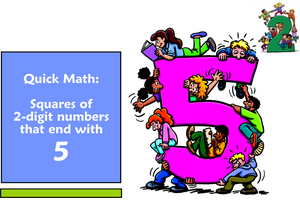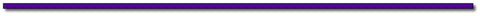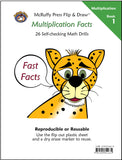# Quick Math: Find the Squares of Numbers That End With 5Want to teach your child some amazing math shortcuts? Follow our new blog series. The first blog features a math trick to quickly square numbers that end with 5.

It’s simple enough for children who have knowledge of basic multiplication facts.

For younger children simply teach the trick. For students learning algebra, explore in the Why it Works section for the more mathematically curious. In future blogs, we’ll do some interesting things with this trick to multiply numbers that aren’t squares.

The Trick:

You can quickly find the square of any two digit number ending with 5, such as 15, 25, 35, 45, etc. The trick actually works for any number that ends with five, but the mental math becomes increasingly difficult with numbers greater than 95.

Simply look at the digit in the tens place and multiply by the next digit. The product will be the digits for the thousands and hundreds place (Note the thousands place will be zero for 152 and 252). Add 25 to that product for the product of the number squared.

For example: 352

Multiply the number in the tens place, 3 by the next number, 4.

3 x 4 = 12 the product is the thousands and hundreds place digits. The 12 represents 1200. Then add 25:

352 = 1225

Print Teaching Sheets for the trick. On the first two sheets ask students to put in a random digit in the first box to choose a number to square. Students use that digit and that digit + 1 to make a multiplication problem for the thousands and hundreds place values. If the product is a single digit, the thousands place is left blank (152 and 252). The third page is a practice sheet for the numbers 15 to 95.

Why it works:

Think of any square of a two digit number as the square of a sum of the tens and ones places.

Let’s make the tens place X and the units (ones) place Y.

So, for the number 35,  x = 30 and y = 5

The square of 35 is the square of our x and y values. Let’s work with the variables first.

(x + y)2 = (x + y)(x + y) = x2 + xy + xy + y2 = x2 + 2xy + y2

When there are 5 ones (y = 5) then 2xy is 10x .

So the problem simplifies to x2 + 10x + y2   Furthermore, y2 will be 52 = 25

So, x2 + 10x + 25

We can group using the associative property and factor:  (x2 +10x) + 25  = x(x +10) + 25

X+10 is the current tens place plus ten more, which is the next ten. In our example, it will be 30 and ten more, 40. So the product of X times the next ten in our example is 1200.

Add 25 to 1200 in our example of 352 = 1200 + 25 = 1225.

Try it with all the squares that end with 5 from 15 to 95.

152 = 225
252 = 625
352 = 1225
452 = 2025
552 = 3025
652 = 4225
752 = 5625
852 = 7225
952 = 9025

You may continue forever, but the mental math becomes more cumbersome. For 1052 The product of 10 x 11 generates the ten thousands, thousands, and hundreds places. Tens and Units will still be 25

1052 = 11025
1152 = 13225## Thanks for Visting Free Gift:

Offer Expires June 30, 2017Subscribe to this blog's RSS feed using http://feeds.feedburner.com/McruffyPress-McruffyMathBlog## McRuffy Press offers complete math curriculum by grade level, math activity books, manipulatives, games and more! Find more McRuffy Math products here.

Improve multiplication facts skills with our Multiplication Fast Fact books:Multiplication Facts (Book 1) Drills factors from 1 to 10 in our self-checking, non-consumable format that allows students to practice until they have mastered basic facts.Multiplication Practice (Book 2) Drills factors from 1 to 10 in our self-checking, non-consumable format that allows students to practice until they have mastered basic facts.